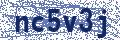# Funny Status Message #66705

Funny Status Messages | Status Message Generator | Recent Comments
X is If you purchased \$1000 worth of Delta airlines stock one year ago, you would have \$49. If you invested it in aig you'd have \$33. If you spent \$1000 on beer and recycled the cans you would have \$214.50. Therefor drinking heavily is your best investment.Save as Image
Related Status Messages:
• # 66586 purchased \$1,000 worth of beer, drank all the beer, turned in the aluminum cans for recycling, you would have \$214.00. Therefore, the best current investment plan is to drink heavily & recycle. It is called the 401-Keg Plan!
• # 113826 found the secret to life via this mathematical formula. To calculate, enter the following in Google: exp((-(((x-4)^2+(y-4)^2)^2))/1000)+exp((-(((x+4)^2+(y+4)^2)^2))/1000)+0.15*exp(-(((x+4)^2+(y+ 4)^2)^2))+0.15*exp(-(((x-4)^2+(y-4)^2)^2
• # 135633 If you cut soda from your diet, you'll save over \$1000 a year and could spend money on more important things, like beer, meth, and skittles.
←Rate | 08-09-2011 11:39 by Will | Tags: Approved
 Post a Comment: Comment: Name:Security Code: